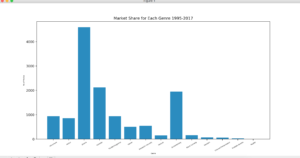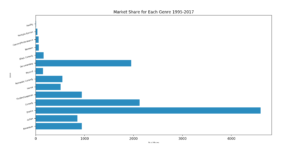In last post I covered line graph. In this post I am going to show how to draw bar graph by using Matplotlib.

## Bar Graph

What is bar graph? According to Wikipedia

bar chart or bar graph is a chart or graph that presents categorical data with rectangular bars with heights or lengths proportional to the values that they represent. The bars can be plotted vertically or horizontally.

So in short, bar graphs are good if you to want to present the data of different groups that are being compared with each other.

For this post I have picked the data of movies genres released from 1995-2017. The source of data is here.

```import matplotlib.pyplot as plt
import numpy as np

label = ['Adventure', 'Action', 'Drama', 'Comedy', 'Thriller/Suspense', 'Horror', 'Romantic Comedy', 'Musical',
'Documentary', 'Black Comedy', 'Western', 'Concert/Performance', 'Multiple Genres', 'Reality']

no_movies = [
941,
854,
4595,
2125,
942,
509,
548,
149,
1952,
161,
64,
61,
35,
5
]```

I take two lists, `label` and `no_movies` and put the respective data into it.

`index = np.arange(len(label))`

`arange` is `numpy`method that generates an array of sequential numbers. For instance `numpy.arange(5)` will generate a `numpy.ndArray` like `[0,1,2,3,4,5]`

Why is it needed? We need some data for X-axis and right now we only have labels that can’t be used for plotting purpose. So we will generate an array of length of `label` and use it on X-axis. Code for the graph is given below:

```def plot_bar_x():
# this is for plotting purpose
index = np.arange(len(label))
plt.bar(index, no_movies)
plt.xlabel('Genre', fontsize=5)
plt.ylabel('No of Movies', fontsize=5)
plt.xticks(index, label, fontsize=5, rotation=30)
plt.title('Market Share for Each Genre 1995-2017')
plt.show()```

you see for `xticks` I used both `index` and `label`. Labels will be placed on each tick that is generated due to `index` sequence. I also give a bit of room for x-Axis labels by rotating them. When run something like below will be generated.You can also generate a horizontal bar graph. You will need to use `barh` method.The graph tells that more movies released in Drama genre than Comedy. Though it should be inverse as the world more happy and positive things than sad/emotional one.

That’s it for now. In coming post I will be covering histogram graph.

## If you like this post then you should subscribe to my blog for future updates.

* indicates required